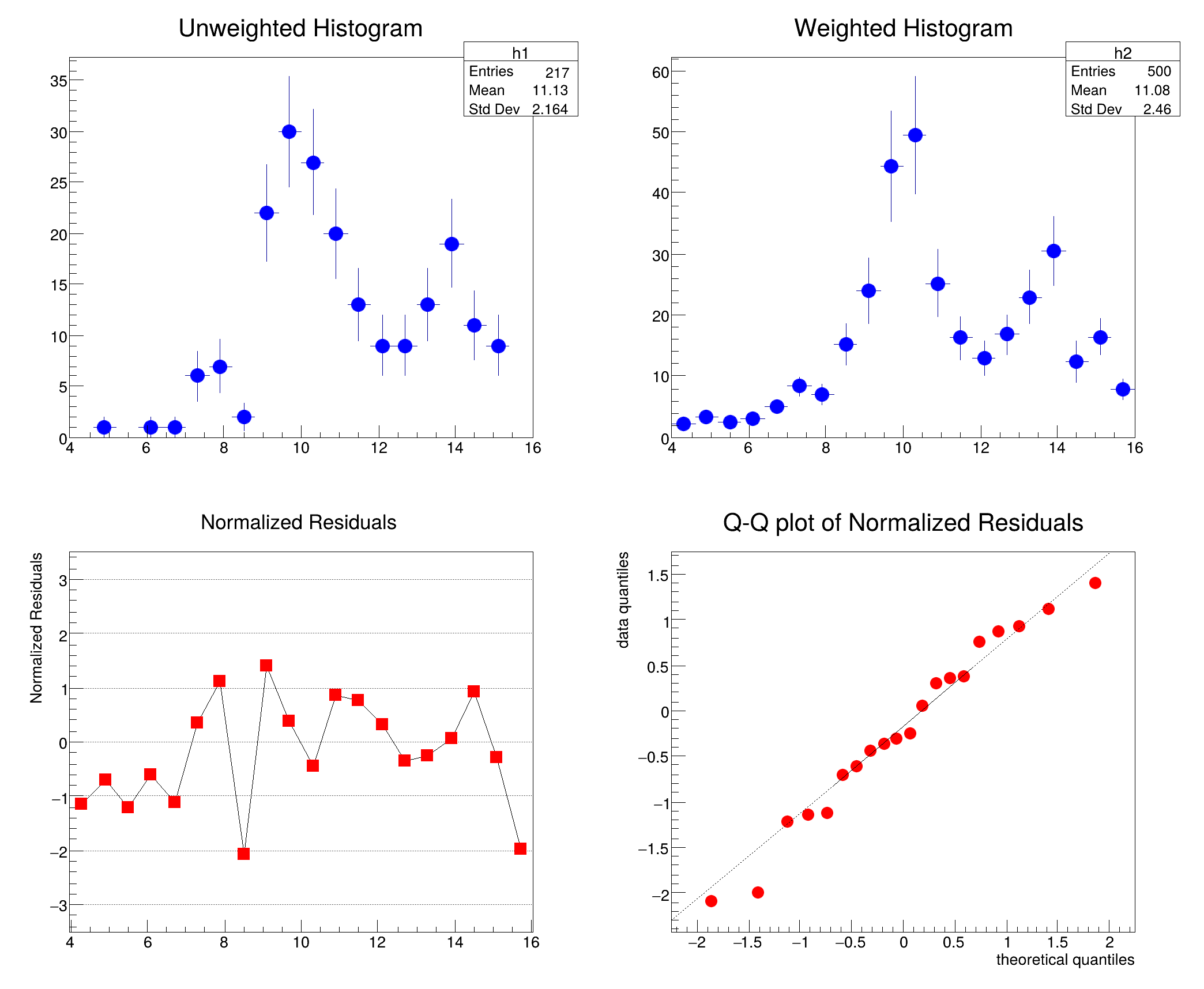ROOT   Reference Guidechi2test.C File Reference

## Detailed DescriptionExample to use chi2 test for comparing two histograms One unweighted histogram is compared with a weighted histogram.

The normalized residuals are retrieved and plotted in a simple graph. The QQ plot of the normalized residual using the normal distribution is also plotted.Chi2 = 21.085124, Prob = 0.332116, NDF = 19, igood = 1
(TCanvas *) 0x55ba6e6ee7e0
#include "TH1.h"
#include "TH1D.h"
#include "TF1.h"
#include "TGraph.h"
#include "TGraphQQ.h"
#include "TCanvas.h"
#include "TStyle.h"
#include "TMath.h"
TCanvas * chi2test(Float_t w=0)
{
// Note: The parameter w is used to produce the 2 pictures in
// the TH1::Chi2Test method. The 1st picture is produced with
// w=0 and the 2nd with w=17 (see TH1::Chi2Test() help).
// Define Histograms.
const Int_t n = 20;
TH1D *h1 = new TH1D("h1", "h1", n, 4, 16);
TH1D *h2 = new TH1D("h2", "h2", n, 4, 16);
h1->SetTitle("Unweighted Histogram");
h2->SetTitle("Weighted Histogram");
h1->SetBinContent(1, 0);
h1->SetBinContent(2, 1);
h1->SetBinContent(3, 0);
h1->SetBinContent(4, 1);
h1->SetBinContent(5, 1);
h1->SetBinContent(6, 6);
h1->SetBinContent(7, 7);
h1->SetBinContent(8, 2);
h1->SetBinContent(9, 22);
h1->SetBinContent(10, 30);
h1->SetBinContent(11, 27);
h1->SetBinContent(12, 20);
h1->SetBinContent(13, 13);
h1->SetBinContent(14, 9);
h1->SetBinContent(15, 9 + w);
h1->SetBinContent(16, 13);
h1->SetBinContent(17, 19);
h1->SetBinContent(18, 11);
h1->SetBinContent(19, 9);
h1->SetBinContent(20, 0);
h2->SetBinContent(1, 2.20173025 );
h2->SetBinContent(2, 3.30143857);
h2->SetBinContent(3, 2.5892849);
h2->SetBinContent(4, 2.99990201);
h2->SetBinContent(5, 4.92877054);
h2->SetBinContent(6, 8.33036995);
h2->SetBinContent(7, 6.95084763);
h2->SetBinContent(8, 15.206357);
h2->SetBinContent(9, 23.9236012);
h2->SetBinContent(10, 44.3848114);
h2->SetBinContent(11, 49.4465599);
h2->SetBinContent(12, 25.1868858);
h2->SetBinContent(13, 16.3129692);
h2->SetBinContent(14, 13.0289612);
h2->SetBinContent(15, 16.7857609);
h2->SetBinContent(16, 22.9914703);
h2->SetBinContent(17, 30.5279255);
h2->SetBinContent(18, 12.5252123);
h2->SetBinContent(19, 16.4104557);
h2->SetBinContent(20, 7.86067867);
h2->SetBinError(1, 0.38974303 );
h2->SetBinError(2, 0.536510944);
h2->SetBinError(3, 0.529702604);
h2->SetBinError(4, 0.642001867);
h2->SetBinError(5, 0.969341516);
h2->SetBinError(6, 1.47611344);
h2->SetBinError(7, 1.69797957);
h2->SetBinError(8, 3.28577447);
h2->SetBinError(9, 5.40784931);
h2->SetBinError(10, 9.10106468);
h2->SetBinError(11, 9.73541737);
h2->SetBinError(12, 5.55019951);
h2->SetBinError(13, 3.57914758);
h2->SetBinError(14, 2.77877331);
h2->SetBinError(15, 3.23697519);
h2->SetBinError(16, 4.3608489);
h2->SetBinError(17, 5.77172089);
h2->SetBinError(18, 3.38666105);
h2->SetBinError(19, 2.98861837);
h2->SetBinError(20, 1.58402085);
h1->SetEntries(217);
h2->SetEntries(500);
//apply the chi2 test and retrieve the residuals
Double_t res[n], x;
h1->Chi2Test(h2,"UW P",res);
//Graph for Residuals
for (Int_t i=0; i<n; i++) x[i]= 4.+i*12./20.+12./40.;
TGraph *resgr = new TGraph(n,x,res);
resgr->GetXaxis()->SetRangeUser(4,16);
resgr->GetYaxis()->SetRangeUser(-3.5,3.5);
resgr->GetYaxis()->SetTitle("Normalized Residuals");
resgr->SetMarkerStyle(21);
resgr->SetMarkerColor(2);
resgr->SetMarkerSize(.9);
resgr->SetTitle("Normalized Residuals");
//Quantile-Quantile plot
TF1 *f = new TF1("f","TMath::Gaus(x,0,1)",-10,10);
TGraphQQ *qqplot = new TGraphQQ(n,res,f);
qqplot->SetMarkerStyle(20);
qqplot->SetMarkerColor(2);
qqplot->SetMarkerSize(.9);
qqplot->SetTitle("Q-Q plot of Normalized Residuals");
//create Canvas
TCanvas *c1 = new TCanvas("c1","Chistat Plot",10,10,700,600);
c1->Divide(2,2);
// Draw Histogramms and Graphs
c1->cd(1);
h1->Draw("E");
c1->cd(2);
h2->Draw("");
h2->SetMarkerStyle(20);
c1->cd(3);
resgr->Draw("APL");
c1->cd(4);
qqplot->Draw("AP");
c1->cd(0);
c1->Update();
return c1;
}
#define f(i)
Definition: RSha256.hxx:104
int Int_t
Definition: RtypesCore.h:45
float Float_t
Definition: RtypesCore.h:57
double Double_t
Definition: RtypesCore.h:59
virtual void SetMarkerColor(Color_t mcolor=1)
Set the marker color.
Definition: TAttMarker.h:38
virtual void SetMarkerStyle(Style_t mstyle=1)
Set the marker style.
Definition: TAttMarker.h:40
virtual void SetMarkerSize(Size_t msize=1)
Set the marker size.
Definition: TAttMarker.h:41
virtual void SetRangeUser(Double_t ufirst, Double_t ulast)
Set the viewing range for the axis from ufirst to ulast (in user coordinates, that is,...
Definition: TAxis.cxx:973
The Canvas class.
Definition: TCanvas.h:23
1-Dim function class
Definition: TF1.h:213
This class allows to draw quantile-quantile plots.
Definition: TGraphQQ.h:18
A TGraph is an object made of two arrays X and Y with npoints each.
Definition: TGraph.h:41
void Draw(Option_t *chopt="") override
Draw this graph with its current attributes.
Definition: TGraph.cxx:806
TAxis * GetXaxis() const
Get x axis of the graph.
Definition: TGraph.cxx:1551
TAxis * GetYaxis() const
Get y axis of the graph.
Definition: TGraph.cxx:1561
void SetTitle(const char *title="") override
Change (i.e.
Definition: TGraph.cxx:2336
1-D histogram with a double per channel (see TH1 documentation)}
Definition: TH1.h:617
void SetTitle(const char *title) override
Definition: TH1.cxx:6710
virtual Double_t Chi2Test(const TH1 *h2, Option_t *option="UU", Double_t *res=0) const
test for comparing weighted and unweighted histograms
Definition: TH1.cxx:2002
virtual void SetBinError(Int_t bin, Double_t error)
Set the bin Error Note that this resets the bin eror option to be of Normal Type and for the non-empt...
Definition: TH1.cxx:9083
void Draw(Option_t *option="") override
Draw this histogram with options.
Definition: TH1.cxx:3070
virtual void SetBinContent(Int_t bin, Double_t content)
Set bin content see convention for numbering bins in TH1::GetBin In case the bin number is greater th...
Definition: TH1.cxx:9099
virtual void SetEntries(Double_t n)
Definition: TH1.h:384
virtual void SetTitle(const char *title="")
Set the title of the TNamed.
Definition: TNamed.cxx:164
return c1
Definition: legend1.C:41
Double_t x[n]
Definition: legend1.C:17
const Int_t n
Definition: legend1.C:16
TH1F * h1
Definition: legend1.C:5

Definition in file chi2test.C.